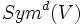# Molien series

This term makes sense in the context of a linear representation of a group, viz an action of the group as linear automorphisms of a vector space

## Definition

Let$G$ be a group and$\rho:G \to GL(V)$ be a finite-dimensional linear representation of$G$. The Molien series of$\rho$ is the formal power series:$\sum n_d t^d$

where$n_d$ is the dimension of the subspace of$Sym^d(V)$ comprising those elements that are invariant under the action of$G$.

Equivalently, the map$\rho:G \to GL(V)$ gives an action of$G$ on the polynomial ring in$dim(V)$ variables. The dimension of the space of homogeneous degree$d$ polynomials that are invariant under this action, is the coefficient$n_d$ of$t^d$.

## Facts

For a finite group, the Molien series corresponding to any finite-dimensional representation is a rational function of the formal variable$t$.